Publicité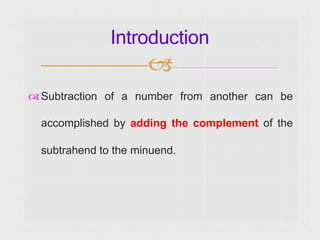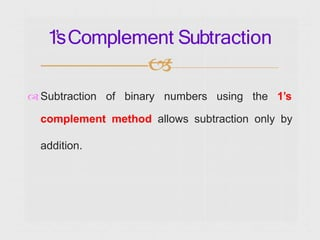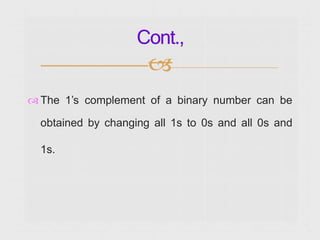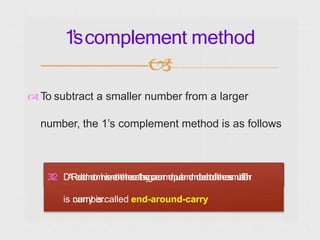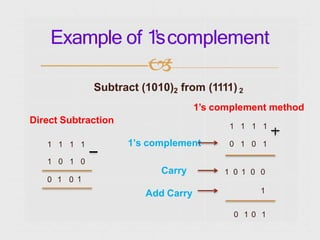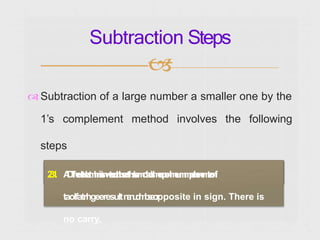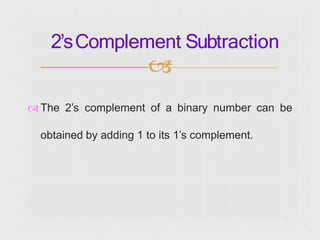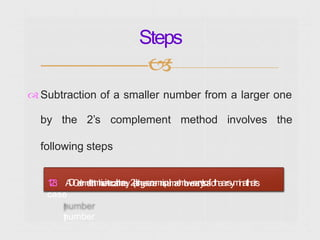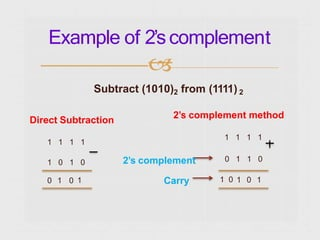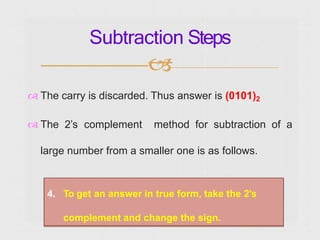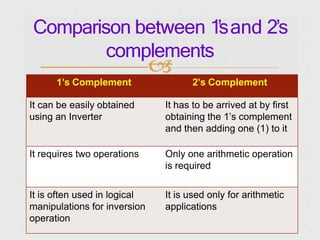1 sur 13
Publicité

### 1sand2scomplement.pptx

1.  Subtraction of a number from another can be accomplished by adding the complement of the subtrahend to the minuend. Introduction 
2. 1’sComplement Subtraction   Subtraction of binary numbers using the 1’s complement method allows subtraction only by addition.
3.  The 1’s complement of a binary number can be obtained by changing all 1s to 0s and all 0s and 1s. Cont., 
4.  To subtract a smaller number from a larger number, the 1’s complement method is as follows 1’scomplement method  3 1 2 . D A R e d e t d m e r t o m h v i i s e n e t t o h t e h t h e c e a 1 l r ’ a s r y r g c a e o n r m d n p u a l e m d m d b e e i t n r t t o o t f h t e h e r e s s m u l a t . l l T e h r is c na ur m ry bi e sr.called end-around-carry
5. Example of 1’scomplement  Direct Subtraction 0 1 0 1 Subtract (1010)2 from (1111) 2 1’s complement method 1 1 1 1 1 1 1 1 1’s complement 0 1 0 1 1 0 1 0 Carry 1 0 1 0 0 Add Carry 1 0 1 0 1
6.  Subtraction of a large number a smaller one by the 1’s complement method involves the following steps Subtraction Steps  2 3 1 . A D T h e d e t d e a r t m h n i s i s n w t e e o r t h t i h s e e t 1 h s ’ s e m c 1 a o ’ l s l m e c r p o n l m e u m m p e l b e n e m t r e o n f taolfatrhgeeresultn a n u d m i b s e o r pposite in sign. There is no carry.
7.  The 2’s complement of a binary number can be obtained by adding 1 to its 1’s complement. 2’sComplement Subtraction 
8.  Subtraction of a smaller number from a larger one by the 2’s complement method involves the following steps Steps  1 2 3 . A D O d e m d t e i t t r h t m h i s e i n t e c o a t t r h h r e e y2 ( l a ’ t s r h g e c e r o r e m n i s p u l m a e l m b w e e a r n y t s o a fcthaerrsyminatllh eirs case n ) umber
9. Example of 2’scomplement  Subtract (1010)2 from (1111) 2 Direct Subtraction 1 1 1 1 1 0 1 0 0 1 0 1 2’s complement method 1 1 1 1 2’s complement Carry 0 1 1 0 1 0 1 0 1
10.  The carry is discarded. Thus answer is (0101)2  The 2’s complement method for subtraction of a large number from a smaller one is as follows. Subtraction Steps  1. Determine the 2’s complement of the larger 2. Add the 2’s complement to the smaller number number 3. There is no carry . The result is in 2’s complement form is negative 4. To get an answer in true form, take the 2’s complement and change the sign.
11.  1’s Complement 2’s Complement It can be easily obtained using an Inverter It has to be arrived at by first obtaining the 1’s complement and then adding one (1) to it It requires two operations Only one arithmetic operation is required It is often used in logical manipulations for inversion operation It is used only for arithmetic applications Comparison between 1’sand 2’s complements
12. ….. Thank You …… The End 
Publicité